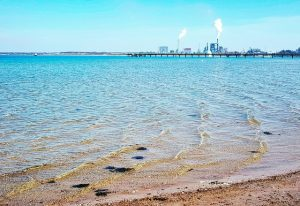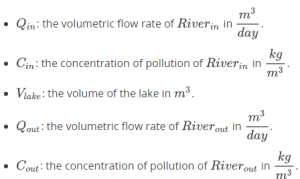# 1.7 Problem

Course subject(s) 1. Introducing Mathematical Modelling

In this practice problem you derive a differential equation for yet another problem. This time, we do not specify the values of the different quantities, so the model and the results stay general. First, the problem.

### Mixing problemA small river, the Riverin, feeds into a lake and at the other side of the lake, the water flows through into another river, the Riverout, that flows on to the sea. The water of the Riverin contains a certain pollutant. A factory along the lake shore is planning to dump more of the same pollutant into the lake.

How much would the factory contribute to the pollution of the lake? Does it add only a few percent, or much more?

### Quantifying time and amount of pollutant

The amount of pollution can be quantified in various ways: in kg, in mol, in a concentration of either…. Here we will simply choose kg. An appropriate unit for the time t is days. So we define:

t: time, with [t]= day.

Z(t): the total mass of the pollution dissolved in the lake, with [Z]= kg.

We assume that the water in the lake mixes well, so the pollution of the factory is distributed quickly over the lake. With quickly, we do not actually mean in a nano-second, but say, in half an hour or so: much quicker than in a day, so we can assume that the water in the lake is ideally mixed, i.e. on the timescale of the mathematical model, instantaniously.

First, we define the quantities for the river feeding into the lake, for the lake itself, and for the river flowing out of the lake: